# Catch up on the latest AI articles# Highly Accurate Image Classification Without Sharing Raw Data! Introducing Model-Contrastive Federated Learning

3 main points
✔️ Introducing contrast learning in coalition learning enables highly accurate image classification
✔️ Introducing SimCLR, which leverages data augmentation in contrast learning
✔️ Improving accuracy by introducing not only image-to-image comparisons but also model-to-model output comparisons

Model-Contrastive Federated Learning
written by Qinbin LiBingsheng HeDawn Song
(Submitted on 30 Mar 2021)

Subjects:  Machine Learning (cs.LG); Artificial Intelligence (cs.AI); Computer Vision and Pattern Recognition (cs.CV)code：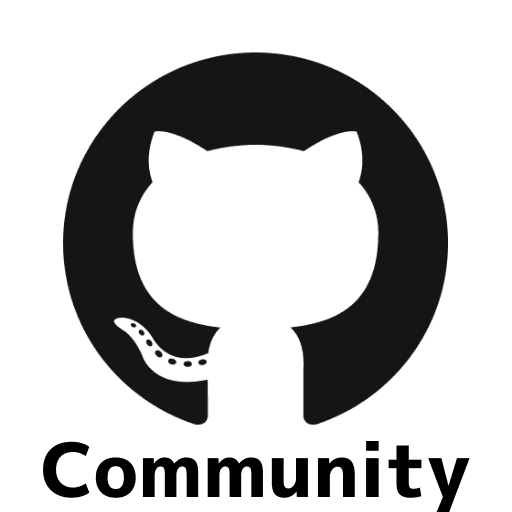The images used in this article are from the paper, the introductory slides, or were created based on them.

## Coalition Learning Overview

Coalitional learning is a method of developing machine learning models by preparing machine learning models for each of those clients or models, training them, and then sharing the resulting updated results (differences in weights) for each model under situations where the data set is distributed across multiple clients or devices. This is a method of model development.

### How Coalition Learning was introduced

Prior to the advent of federative learning, when data was dispersed, it was necessary to aggregate the data on a central server prior to learning.

However, because conventional methods require each medium to send raw data to a central server, there is a risk of information leakage when handling data that contains personal or confidential information. In addition, the central server, where data is concentrated in a single location, is vulnerable to attacks.

Furthermore, as people's awareness of privacy has increased in recent years, an increasing number of companies and organizations have become resistant to the traditional method of aggregating data and then performing machine learning. Under these circumstances, a method called federative learning has been proposed.

Coalitional learning is a method that allows machine learning models to be developed without having to share distributed data itself, and has attracted a great deal of attention in recent years.

### Types of Coalitional Learning (Horizontal Coalitional Learning and Vertical Coalitional Learning)

Coalitional learning can be classified into horizontal andvertical coalitional learning based on the criterion of which parts of the data that multiple media have in common.

Horizontal coalition learning is a method of coalition learning that is used when the data is such that several different media share common features. For example, among the attributes of patients in different hospitals, basic elements such as age and gender are considered to be common to all hospitals. Therefore, horizontal coalition learning is used to integrate these data.

Vertical Association Learning, on the other hand, is a method used when data from several different media have a common target. For example, a user belongs to a hospital and a financial institution, and vertical association learning is used to integrate their data.

Since horizontal coalition learning is introduced in this paper, this article describes horizontal coalition learning in detail.

### Algorithms for Coalitional Learning

As mentioned above, horizontal association learning is a model used to integrate data that are distributed across multiple organizations and share common features. The algorithm for horizontal coalition learning is as follows.

Step0: Fix the parameters of the model owned by the central server (this model is called the global model ) to a random state.

Step 1: The global model owned by the central server is distributed to each client participating in the federated learning.

Step2: Each client learns using the distributed model with its own local data.

Step3: Each client sends thedifference in update weights resulting from the updateto the central server.

Step4: The central server receives the local model information collected from each client and updates the global model based on this information.

Steps 1 through 4 are referred to as a single round, and in coalition learning, multiple rounds are repeated to gradually improve the accuracy of the global model.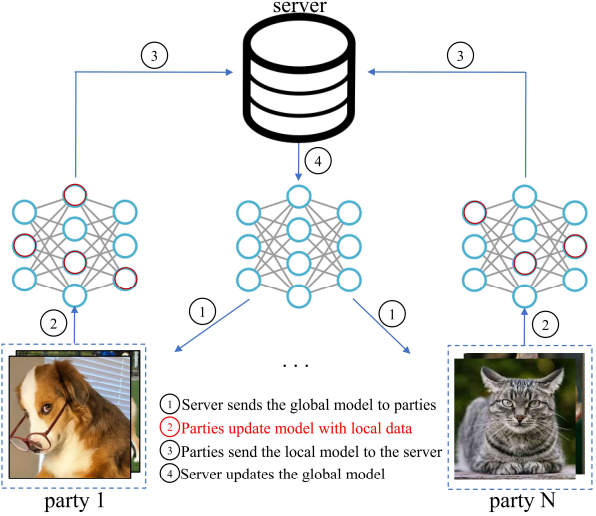In the first paper in which coalition learning was proposed, the global model was determined so that the weighted average of the loss functions collected from each client was minimized. However, this model was not accurate enough for coalition learning when the data possessed by the clients were unbalanced.

For this reason, improvement methods are now being proposed , such as adding a correction term according to the loss function depending on the nature of the data set, rather than simply taking a weighted average of the clients. (MOON, introduced in this paper, is one such improvement method.)

### Application of Coalitional Learning to Image Classification

Coalitional learning is a technique that has been used in areas such as tabular data analysis and text data classification, and is currently being applied to the area of image classification.

However, image data is more complex and diverse than tabular or text data, and these data tend to be unbalanced among clients. Therefore, when coalition learning is applied to image classification, the global model and the local model may diverge, resulting in inaccuracies.

Therefore, in order to solve those problems, there exists a method of introducing contrastive learning as a pre-study to the associative learning.

## Contrastive Learning Overview

Contrastive learning is an unsupervised learning technique that takes advantage of the fact that images with similar final classification results are similar to each other. The loss function of contrastive learning is such that pairs of images belonging to the same class are more similar and pairs of images belonging to different classes are less similar, and machine learning is performed to minimize this loss function.

In contrast learning, feature vectors obtained by projecting images into the feature space are generally compared, rather than the images themselves. This feature vector is obtained by extracting features from the client's image using a CNN encoder.

CNN is a neural network model specialized for image classification that improves the efficiency of parameters in learning by introducing convolution. It is characterized by a convolutional layer that extracts features and a pooling layer that compresses information and learns to maintain robustness against parallel shifts. AlexNet andGoogleNet are well-known CNN models.

### How Contrastive Learning Works

Contrastive learning is one of the main unsupervised learning methods, among them self-supervised learning.

There are various approaches to unsupervised learning, such as generative, discriminative-regressive, and comparative tasks, etc. Methods classified as discriminative-regressive orcomparative tasks are sometimes called self-supervised learning methods.

As the name "self-supervised" suggests, the method creates a teacher signal (label) from the data itself. This technique is used in image generation with autoencoders and in the process of learning embedded word representations in natural language processing.

Self-supervised learning has a structure that combines prior learning andfine tuning, and has the potential to be applied to a wide variety of tasks while increasing universality in learning. It is expected to be used in various fields such as speech recognition and automatic driving.

### Typical Example of Contrastive Learning (SimCLR) Mechanism

How can we learn the semantic distance between images in the absence of a teacher? In this article, we will explain the key points of contrast learning, using the model SimCLR, a typical example of contrast learning, as an example to unravel the mechanism of contrast learning.

SimCLR is a model of contrast learning in which one particular image is intentionally flipped or rotated so that the distance between images derived from the same image is smaller, and the distance between different images is larger. In other words, it shows that images that are flipped or rotated from the same image are learned so that they can be identified as originating from the same image. Generating a variety of data from a single data set is called data augmentation.

The SimCLR model has become a mainstream model for contrastive learning due to its simplicity and high performance, and is widely used in areas such as natural language processing and image processing. SimCLR models are widely used in areas such as natural language processing and image processing.

SimCLR utilizes the concept of Normalized Temperature Cross Entoropy. The loss function is shown in the following equation.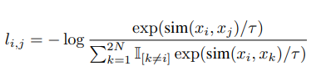Here, sim is a function of cosine similarity, or how similar the feature vectors are in direction.

The numerator shows that the better we learn that the similarity of feature vectors derived from the same image will be larger, the smaller the loss function will be. The denominator computes the cosine similarity of a particular single image xk to all other images except xk, indicating that the better the learning is that the similarity of feature vectors derived from different images is smaller, the smaller the loss function will be.

## Overview of MOON

An overview of Model Contrastive Federated Learning (commonly referred to as MOON ), a model proposed in 2021 that successfully takes the accuracy of this method one step further, will be presented.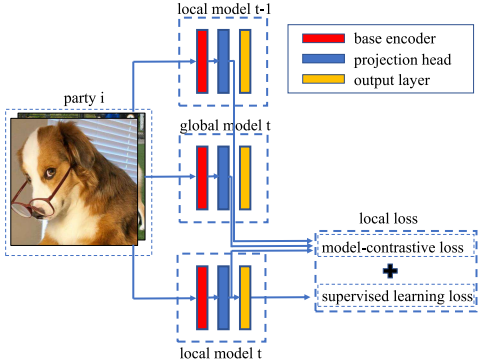The MOON mechanism is illustrated in the figure. Three different machine learning models (from top to bottom: the model at the previous client, the global model, and the current local model ) are run on the image data owned by a client. The outputs are compared.

The red layer is the encoder of the CNN and performs feature extraction. The blue layer is a multi-layer perceptron layer dedicated to representation learning, which converts feature vectors to a certain dimension (256 dimensions in this paper ). The yellow layer represents the probability distribution of the final output classification result. A multilayer perceptron layer is a neural network model that consists of only all coupled layers, and is known for its simplicity and ability to train complex models.

### Definition of the loss function for MOON

As mentioned earlier, in conventional coalition learning for image classification, image classification is performed by introducing contrast learning between images owned by a client (i.e., passing images within a client through a single machine learning model and comparing their outputs). In contrast, the MOON method uses a different approach to derive a loss function and adds it to the conventional loss function.

The specific loss function is as follows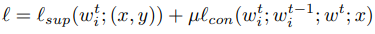μ is a pre-specified hyperparameter. The loss function is the sum of a loss function trained on a single model and a loss function trained on a single image.

In the second half of the equation, we show that for a given image of a particular one, the output obtained through the client's model and the output of the model one round earlier at that client are contrasted and trained.

This is probably the most important part of the model, so I will explain it again using a contrast diagram of the concepts.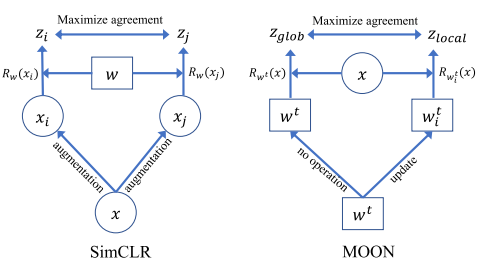The left figure shows the SimCLR method, which runs a single client image through a machine learning model to derive image similarity. The right figure shows the MOON method, which runs a single image through several different machine learning models and compares their outputs. As you can see from this figure, although the composition is similar, we are comparing different concepts.

### How to determine the MOON model

The global model in coalition learning is trained to minimize the loss function by adding the weighted values of each client's average loss function for all clients. The average loss function for each client is the average of the loss functions for all image pairs that exist for that client.

### How the MOON algorithm is determined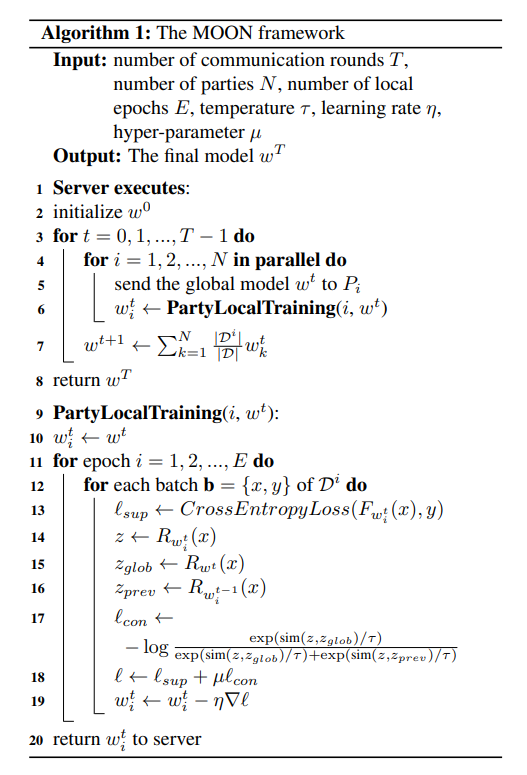The algorithm for updating the model in MOON is shown again in the figure. where T is the total number of communications, N is the total number of clients, E is the number of local epochs, η is the learning rate in coalition learning, and τ is a pre-specified hyperparameter.

## Experimental results

To confirm the accuracy of MOON in image classification, we compared the accuracy of image classification with existing methods such as Fed Average, Fed Prox, and other coalition learning methods. Three image datasets , CIFAR-10, CIFAR-100, and Tiny-ImageNet, were used. All three are benchmark natural image datasets in computer vision.

Res-Net50 is used as the base for image classification. Res-Net is a machine learning model specialized for image classification. It is a model that introduces a mechanism called " skip connections" to solve the problem of de-gradation, in which the deeper the layers, the more difficult it becomes to optimize the function, leading to a loss of accuracy. This concept has been introduced into various deep learning models.

The number of local epochs (the number of virtual clients ) is 10, the number of trials of the experiment is 3, and the mean and standard deviation are derived as follows.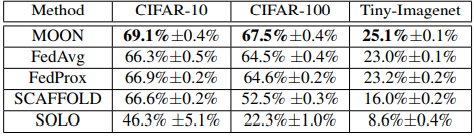The experiments show that MOON achieves higher accuracy in image classification than existing methods on both image datasets.

## Summary and Future Prospects

Coalitional learning is a method that allows machine learning to be performed at low cost while protecting privacy in situations where data is distributed. This method is expected to not only protect privacy, but also reduce the communication cost of sending data to a central server when updating a model.

The ability to update the model in real time is also believed to allow for flexible learning.

In this paper, a model of coalition learning specific to image classification called MOON is proposed, and it is shown that this model significantly outperforms the accuracy of existing coalition learning models.

MOON is expected to be applied to various computer vision applications such as medical image classification and object detection. Furthermore, since MOON is not limited to images as input data, it suggests that the method may be applied to various domains other than visual images. In addition to MOON, methods utilizing self-supervised learning are expected to play an important role in the future, and future research on this topic will be closely watched.

If you have any suggestions for improvement of the content of the article,